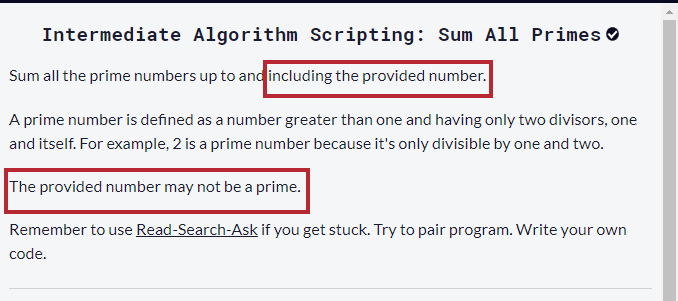# Is the test condition here wrong?

I feel like one of the conditions in this challenge is wrong, shouldn’t sumPrimes(10) be returning 27??

``````
function sumPrimes(num) {
let count = 0;
for(var x = 0; x<num; x++){
let pork = 0;
for(var y = 2; y<x; y++){
if (x % y === 0){
pork += 1;
}
}
if (pork === 0){
count += x;
}
}
console.log(count - 1 + num);
}

sumPrimes(10);

``````

User Agent is: `Mozilla/5.0 (Windows NT 6.3; Win64; x64) AppleWebKit/537.36 (KHTML, like Gecko) Chrome/78.0.3904.108 Safari/537.36`.

Challenge: Sum All Primes

I added this line of code in the meantime.

``````if(num === 10){
count -= 10;
}
``````

Hello!

The challenge says:

Sum all the prime numbers up to and including the provided number

So, if 10 is the input, then the prime numbers between 1 and 10 (inclusive) are:

2, 3, 5 and 7, which equals to 17

If it’s including the provided number wouldn’t it be 2+3+5+7+10(the included number) ??
This was what got me confused, the first condition didnt have the num included whereas the other has it included

Yes, but the inclusive part should be included only when the input is itself a prime. In this case, 10 is not a prime.

If the input number is 11, then that would be included too.

I’m sorry if this isn’t the right way of quoting statements since I’m new to the forums.
It says here to include the number even if it wasn’t primeNo worries, that’s one way to quote.

I don’t understand the instruction like You do.

Since the test expect that when the input is 10 (You can see it below the Run Code buttons), the sum should be 17, which means You have to include the input when it’s itself a prime and exclude it when not.

The instruction says:

The provided number may not be a prime

Which I understand as:

include the number only if it’s prime

Of course, I may be wrong, but taking into account what the test expects, that My interpretation.

I see. I guess the wording made me confused but your approach seems to make more sense. thank you!

1 Like